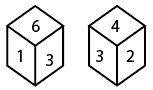Question 7

# Two different positions of the same dice are shown. Which number will be at the top if 6 is at the bottom ?Solution

As we can see from the diagram,

In the first image, 6 and 1 are the adjacent faces of 3 and 4 and 2 are the also the adjacent faces of the 3, So when we will overlap the same faces

On the dice, then we will able to see that the bottom face will be 2 with respect to 6.

• Free SSC Study Material - 18000 Questions
• 230+ SSC previous papers with solutions PDF